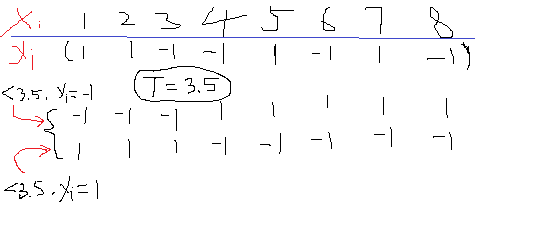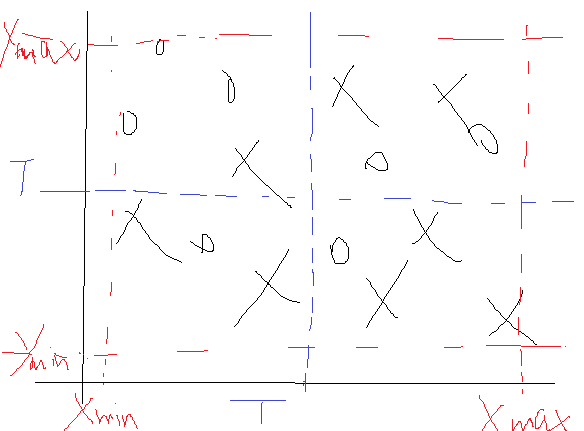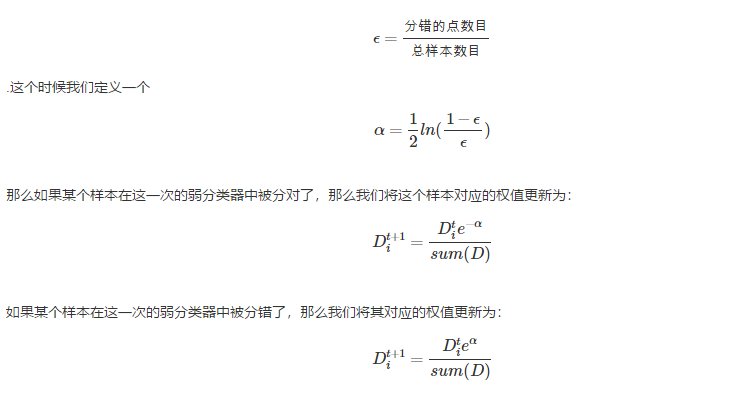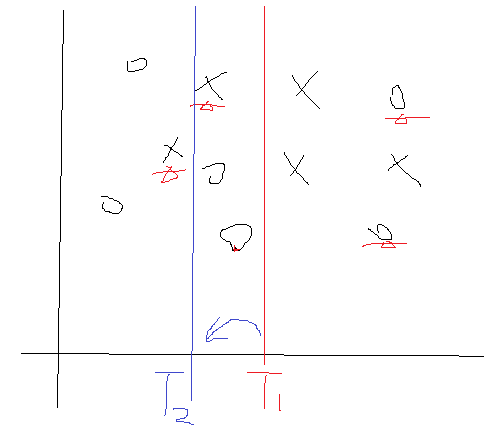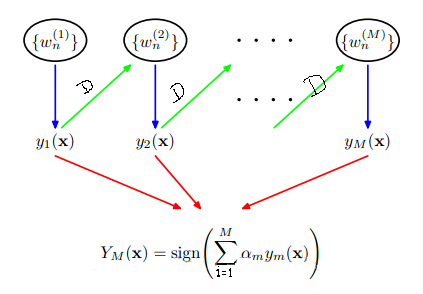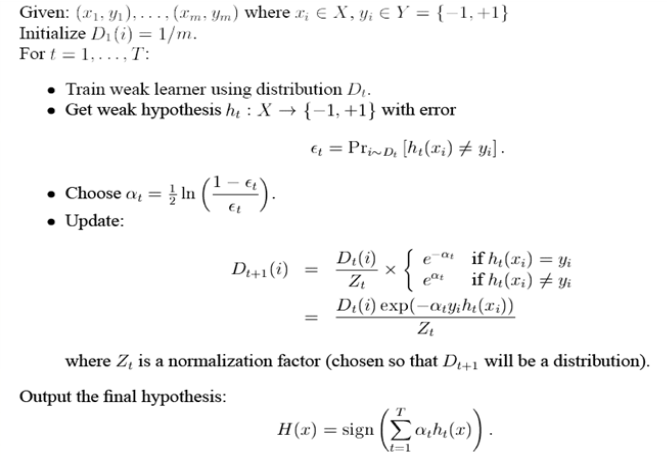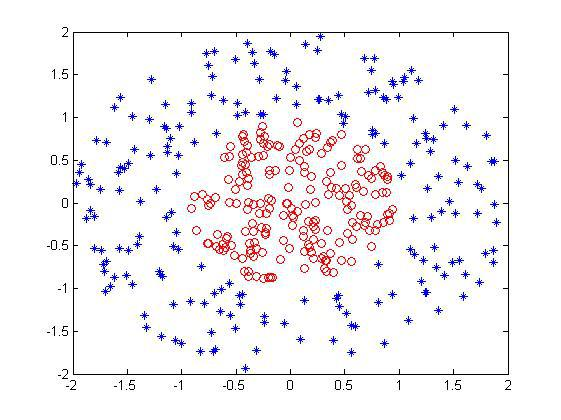function [Dim,Dir,T,best_label,minError] = buildSimpleStump(data,label,D)
% 设置一个步长
numSteps = 50;
% m个样本,每个n维
[m,n] = size(data);
thresh = 0;
minError = inf;
for i = 1:n
min_dataI = min(data(:,i));
max_dataI = max(data(:,i));
for j = 1:numSteps
index = find(data(:,i) <= threshVal);
%-----小于阈值的取值为-1类--------------------
label_temp = ones(m,1);
label_temp(index) = -1;
index1 = find(label_temp == label);
errArr = ones(m,1);
errArr(index1) = 0;
%小于阈值的误差
weightError = D'*errArr;
if weightError < minError
bestLabel = label_temp;
minError = weightError;
%小于阈值的点取-1标签
direction = -1;
Dim = i; %记录属于的维度
thresh = threshVal;
end
%-----------小于阈值的取值为+1类---------
label_temp = -1*ones(m,1);
label_temp(index) = 1;
index1 = find(label_temp == label);
errArr = ones(m,1);
errArr(index1) = 0;
%大于阈值的误差
weightError = D'*errArr;
if weightError < minError
bestLabel = label_temp;
minError = weightError;
%小于阈值的点取+1标签
direction = 1;
Dim = i; %记录属于的维度
thresh = threshVal;
end
end
end
Dir = direction;
T = thresh;
best_label = bestLabel;

function [dim,direction,thresh,alpha] = adaBoostTrainDs(data,label,iter)
[m,~] = size(data);
% 初始化权值D
D = ones(m,1)/m;
alpha = zeros(iter,1);
% 记录T方向
direction = zeros(iter,1);
% 记录T属于哪一个维度
dim = zeros(iter,1);
% 初始化阈值T
thresh = zeros(iter,1);
for i = 1:iter
[dim(i),direction(i),thresh(i),best_label,error] = ...
buildSimpleStump(data,label,D);
%计算alpha
alpha(i) = 0.5*log((1-error)/max(error,1e-15));
%更新权值D
D = D.*(exp(-1*alpha(i)*(label.*best_label)));
D = D/sum(D);
end

clc
clear
close all
%% 加载数据
% * 最终data格式：m*n，m样本数，n维度
% * label:m*1  标签为-1与1这两类
clc
clear
close all
%选择训练样本个数
num_train = 200;
%构造随机选择序列
choose = randperm(length(data));
train_data = data(choose(1:num_train),:);
label_train = train_data(:,end);
train_data = train_data(:,1:end-1);
test_data = data(choose(num_train+1:end),:);
label_test = test_data(:,end);
test_data = test_data(:,1:end-1);
predict = zeros(length(test_data),1);
%% -------训练集训练所有的弱分类器
iter = 50; %规定弱分类器的个数
%% -------预测测试集的样本分类
for i = 1:length(test_data)
data_temp = test_data(i,:);
h = zeros(iter,1);
for j = 1:iter
if direction(j) == -1
if data_temp(dim(j)) <= thresh(j)
h(j) = -1;
else
h(j) = 1;
end
elseif direction(j) == 1
if data_temp(dim(j)) <= thresh(j)
h(j) = 1;
else
h(j) = -1;
end
end
end
predict(i) = sign(alpha'*h);
end
%% 显示结果
figure;
index1 = find(predict==1);
data1 = (test_data(index1,:))';
plot(data1(1,:),data1(2,:),'or');
hold on
index2 = find(predict==-1);
data2 = (test_data(index2,:))';
plot(data2(1,:),data2(2,:),'*');
hold on
indexw = find(predict~=(label_test));
dataw = (test_data(indexw,:))';
plot(dataw(1,:),dataw(2,:),'+g','LineWidth',3);
accuracy = length(find(predict==label_test))/length(test_data);
title(['predict the testing data and the accuracy is :',num2str(accuracy)]);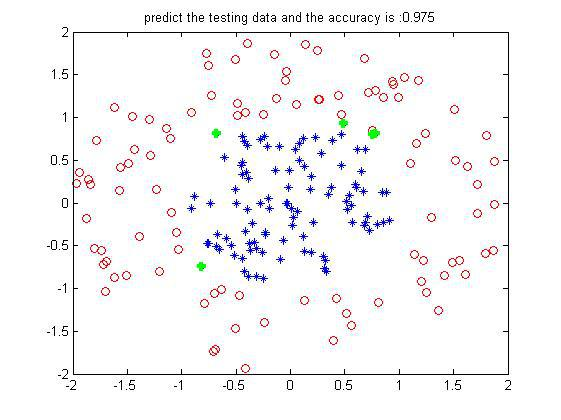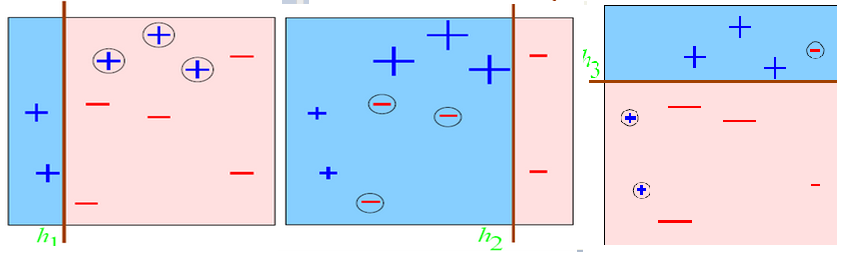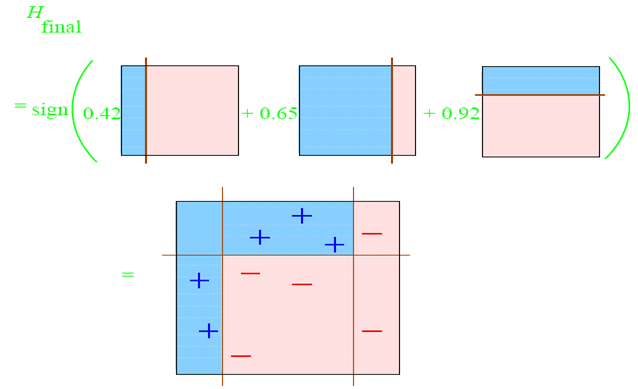----------------------------------------------------这里是分割线-------------------------------------------------------------------------------------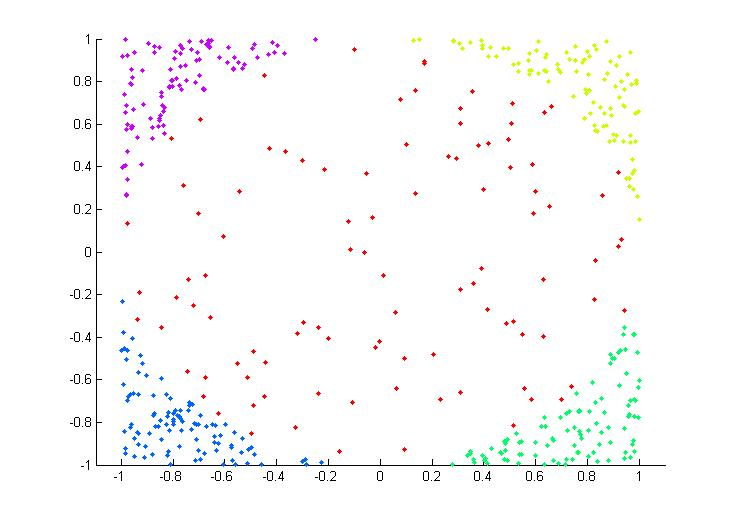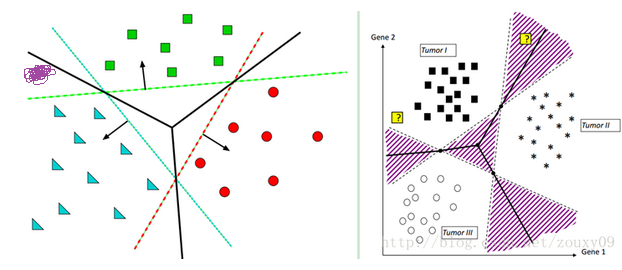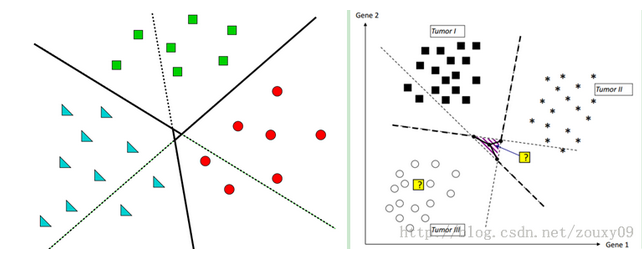function model = adaboost_train(label,data,iter)
[model.dim,model.direction,model.thresh,model.alpha] = ...
model.iter = iter;

function predict = adaboost_predict(data,model)
h = zeros(model.iter,1);
for j = 1:model.iter
if model.direction(j) == -1
if data(model.dim(j)) <= model.thresh(j)
h(j) = -1;
else
h(j) = 1;
end
elseif model.direction(j) == 1
if data(model.dim(j)) <= model.thresh(j)
h(j) = 1;
else
h(j) = -1;
end
end
end
predict = sign(model.alpha'*h);

%%
% * 多类非线性分类
%
%%
clc
clear
close all
% * 数据预处理
num_train = 200;
%构造随机选择序列
choose = randperm(length(data));
train_data = data(choose(1:num_train),:);
gscatter(train_data(:,1),train_data(:,2),train_data(:,3));
label_train = train_data(:,end);
test_data = data(choose(num_train+1:end),:);
label_test = test_data(:,end);
num = 0;
iter = 30;%规定弱分类器的个数
for i = 1:5-1 %5类
for j = i+1:5
num = num + 1;
%重新归类
index1 = find(label_train == i);
index2 = find(label_train == j);
label_temp = zeros((length(index1)+length(index2)),1);
%svm需要将分类标签设置为1与-1
label_temp(1:length(index1)) = 1;
label_temp(length(index1)+1:length(index1)+length(index2)) = -1;
train_temp = [train_data(index1,:);train_data(index2,:)];
% 训练模型
end
end
% 用模型来预测测试集的分类
predict = zeros(length(test_data),1);
for i = 1:length(test_data)
data_test = test_data(i,:);
num = 0;
for j = 1:5-1
for k = j+1:5
num = num + 1;
if temp > 0
else
end
end
end
end
%% show the result--testing
figure;
gscatter(test_data(:,1),test_data(:,2),predict);
accuracy = length(find(predict==label_test))/length(test_data);
title(['predict the testing data and the accuracy is :',num2str(accuracy)]);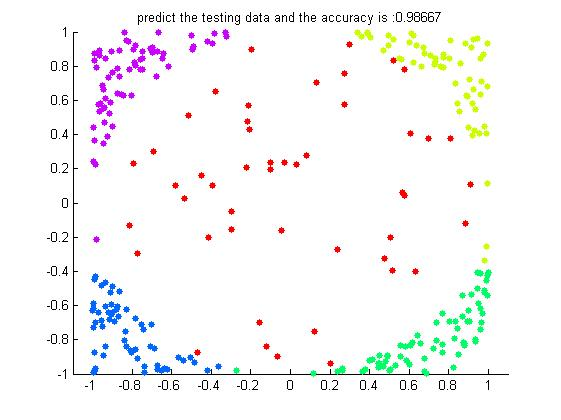%% % * Logistic方法用于回归分析与分类设计 % * 多类问题的分类 % %% 产生非线性数据 2类 200个 clc clear close all data1 = rand(1,1000)*4 + 1; data2 = rand(1,1000)*4 + 1; circle_inx = data1 - 3; circle_iny = data2 - 3; r_in = circle_inx.^2 + circle_iny.^2; index_in = find(r_in<0.8); data_in = [data1(index_in(1:100));data2(index_in(1:100));-1*ones(1,100)]; data1 = rand(1,1000)*4 + 1; data2 = rand(1,1000)*4 + 1; circle_inx = data1 - 3; circle_iny = data2 - 3; r_in = circle_inx.^2 + circle_iny.^2; index_out = find((r_in>1.2)&(r_in<4)); data_out = [data1(index_out(1:100));data2(index_out(1:100));ones(1,100)]; plot(data_in(1,:),data_in(2,:),'or'); hold on; plot(data_out(1,:),data_out(2,:),'*'); data = [data_in,data_out]; save data_test1.mat data

posted @ 2018-07-18 20:11  Horse-Ma  阅读(...)  评论(...编辑  收藏